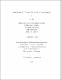## Noncommutative spaces from matrix models2016
##### Publisher
University of Alabama Libraries
##### Abstract

Noncommutative (NC) spaces commonly arise as solutions to matrix model equations of motion. They are natural generalizations of the ordinary commutative spacetime. Such spaces may provide insights into physics close to the Planck scale, where quantum gravity becomes relevant. Although there has been much research in the literature, aspects of these NC spaces need further investigation. In this dissertation, we focus on properties of NC spaces in several different contexts. In particular, we study exact NC spaces which result from solutions to matrix model equations of motion. These spaces are associated with finite-dimensional Lie-algebras. More specifically, they are two-dimensional fuzzy spaces that arise from a three-dimensional Yang-Mills type matrix model, four-dimensional tensor-product fuzzy spaces from a tensorial matrix model, and Snyder algebra from a five-dimensional tensorial matrix model. In the first part of this dissertation, we study two-dimensional NC solutions to matrix equations of motion of extended IKKT-type matrix models in three-space-time dimensions. Perturbations around the NC solutions lead to NC field theories living on a two-dimensional space-time. The commutative limit of the solutions are smooth manifolds which can be associated with closed, open and static two-dimensional cosmologies. One particular solution is a Lorentzian fuzzy sphere, which leads to essentially a fuzzy sphere in the Minkowski space-time. In the commutative limit, this solution leads to an induced metric that does not have a fixed signature, and have a non-constant negative scalar curvature, along with singularities at two fixed latitudes. The singularities are absent in the matrix solution which provides a toy model for resolving the singularities of General relativity. We also discussed the two-dimensional fuzzy de Sitter space-time, which has irreducible representations of su(1,1) Lie-algebra in terms of principal, complementary and discrete series. Field theories on such backgrounds result from perturbations about the solutions. The perturbative analysis requires non-standard Seiberg-Witten maps which depend on the embeddings in the ambient space and the symplectic 2-form. We find interesting properties of the field theories in the commutative limit. For example, stability of the action may require adding symmetry breaking terms to the matrix action, along with a selected range for the matrix coefficients. In the second part of this dissertation, we study higher dimensional fuzzy spaces in a tensorial matrix model, which is a natural generalization to the three-dimensional actions and is valid in any number of space-time dimensions. Four-dimensional tensor product NC spaces can be constructed from two-dimensional NC spaces and may provide a setting for doing four-dimensional NC cosmology. Another solution to the tensorial matrix model equations of motion is the Snyder algebra. A crucial step in exploring NC physics is to understand the structure of the quantized space-time in terms of the group representations of the NC algebra. We therefore study the representation theory of the Snyder algebra and implementation of symmetry transformations on the resulted discrete lattices. We find the three-dimensional Snyder space to be associated with two distinct Hilbert spaces, which define two reducible representations of the su(2)*su(2) algebra. This implies the existence of two distinct lattice structures of Snyder space. The difference between the two representations is evident in the spectra of the position operators, which could only be integers in one case and half integers in the other case. We also show that despite the discrete nature of the Snyder space, continuous translations and rotations can be unitarily implemented on the lattices.

##### Description
Electronic Thesis or Dissertation
##### Keywords
Physics, Theoretical physics